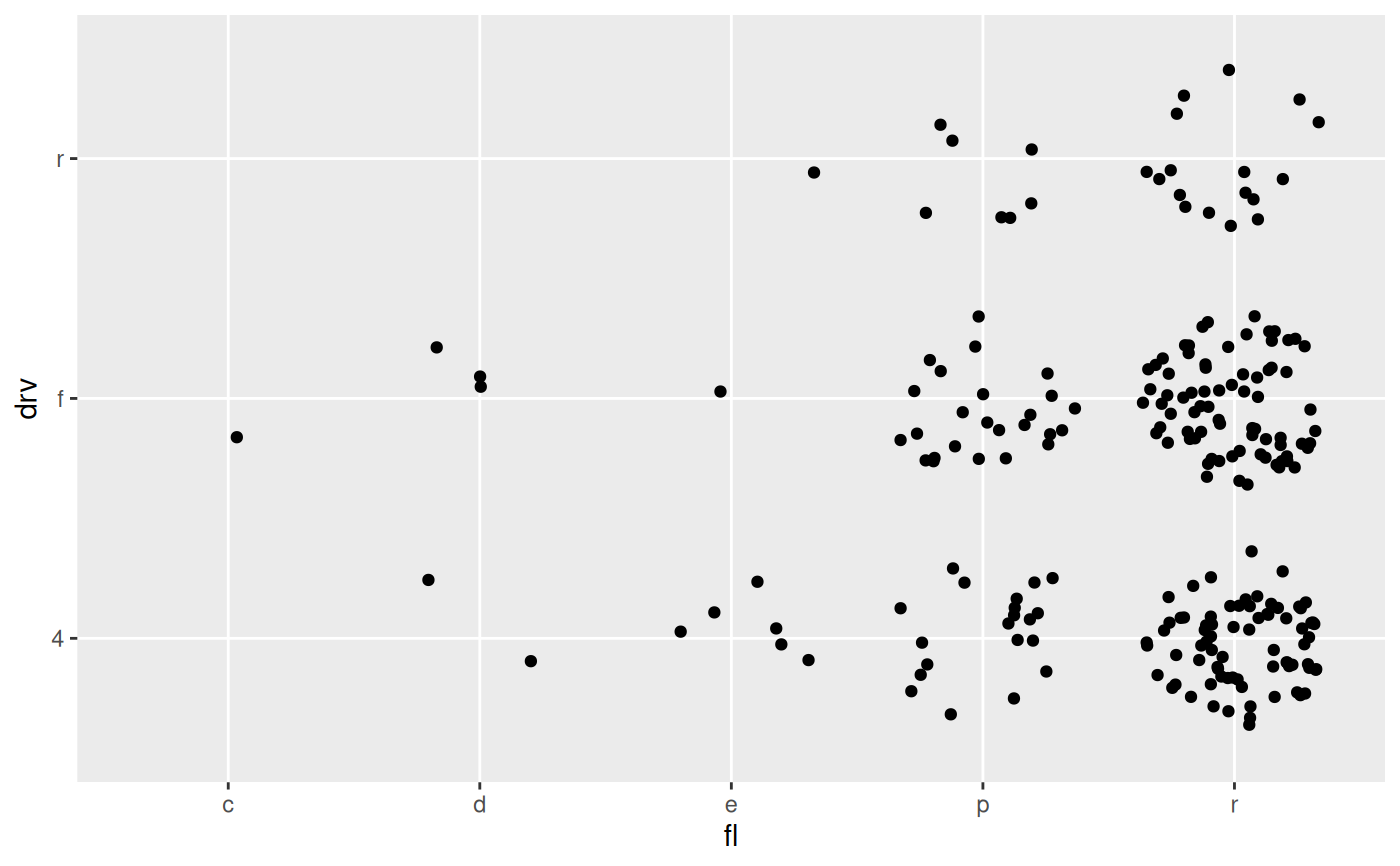This position adjustment is able to select a meaningful jitter of the data based on the combination of positional scale types. IT behaves differently depending on if none, one, or both the x and y scales are discrete. If both are discrete it will jitter the datapoints evenly inside a disc, if one of them is discrete it will jitter the discrete dimension to follow the density along the other dimension (like a sina plot). If neither are discrete it will not do any jittering.

position_auto(jitter.width = 0.75, bw = "nrd0", scale = TRUE, seed = NA)

## Arguments

jitter.width

The maximal width of the jitter

bw

The smoothing bandwidth to use in the case of sina jittering. See the bw argument in stats::density

scale

Should the width of jittering be scaled based on the number of points in the group

seed

A seed to supply to make the jittering reproducible across layers

geom_autopoint for a point geom that uses auto-position by default

## Examples

# Continuous vs continuous: No jitter
ggplot(mpg) + geom_point(aes(cty, hwy), position = 'auto')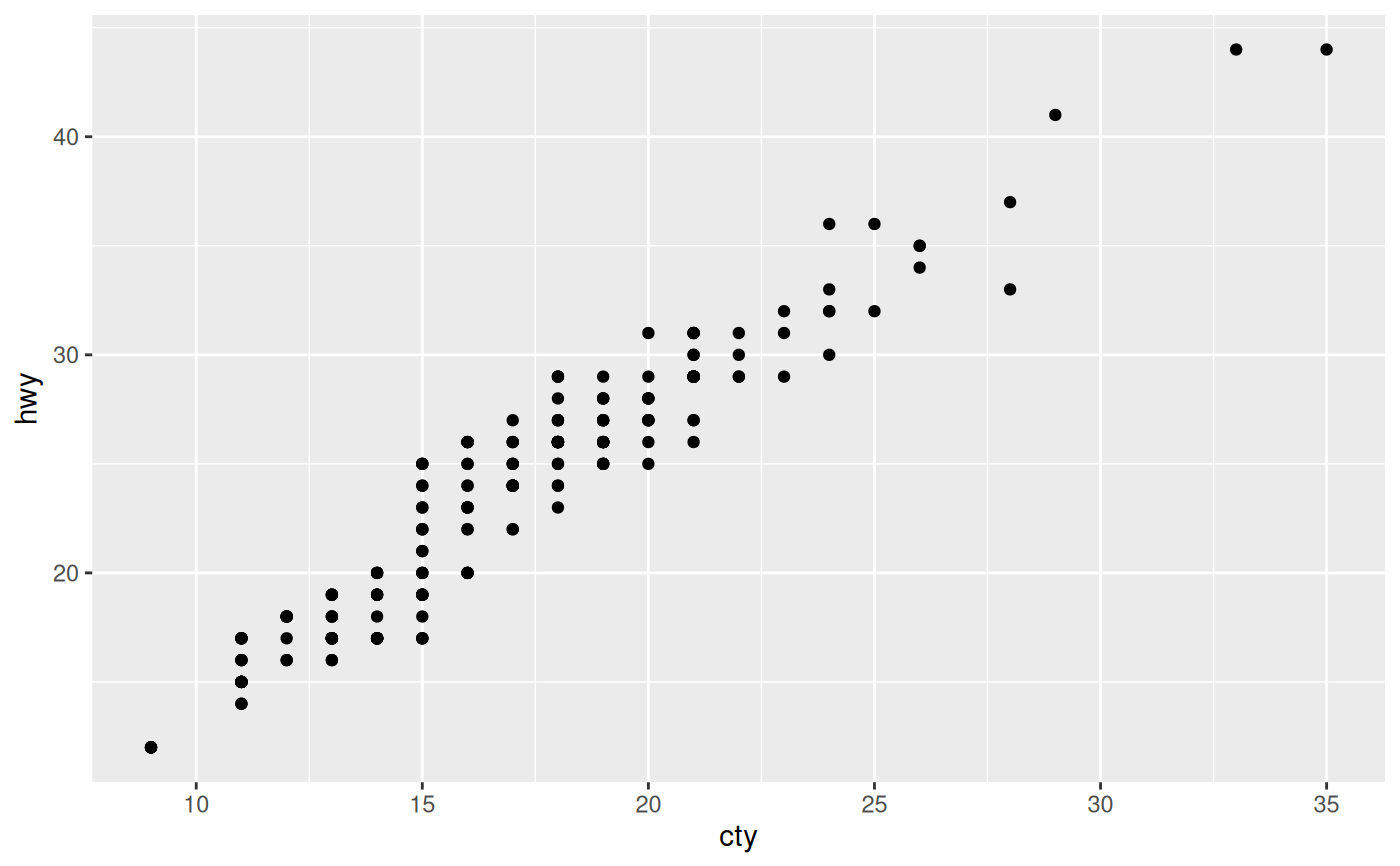# Continuous vs discrete: sina jitter
ggplot(mpg) + geom_point(aes(cty, drv), position = 'auto')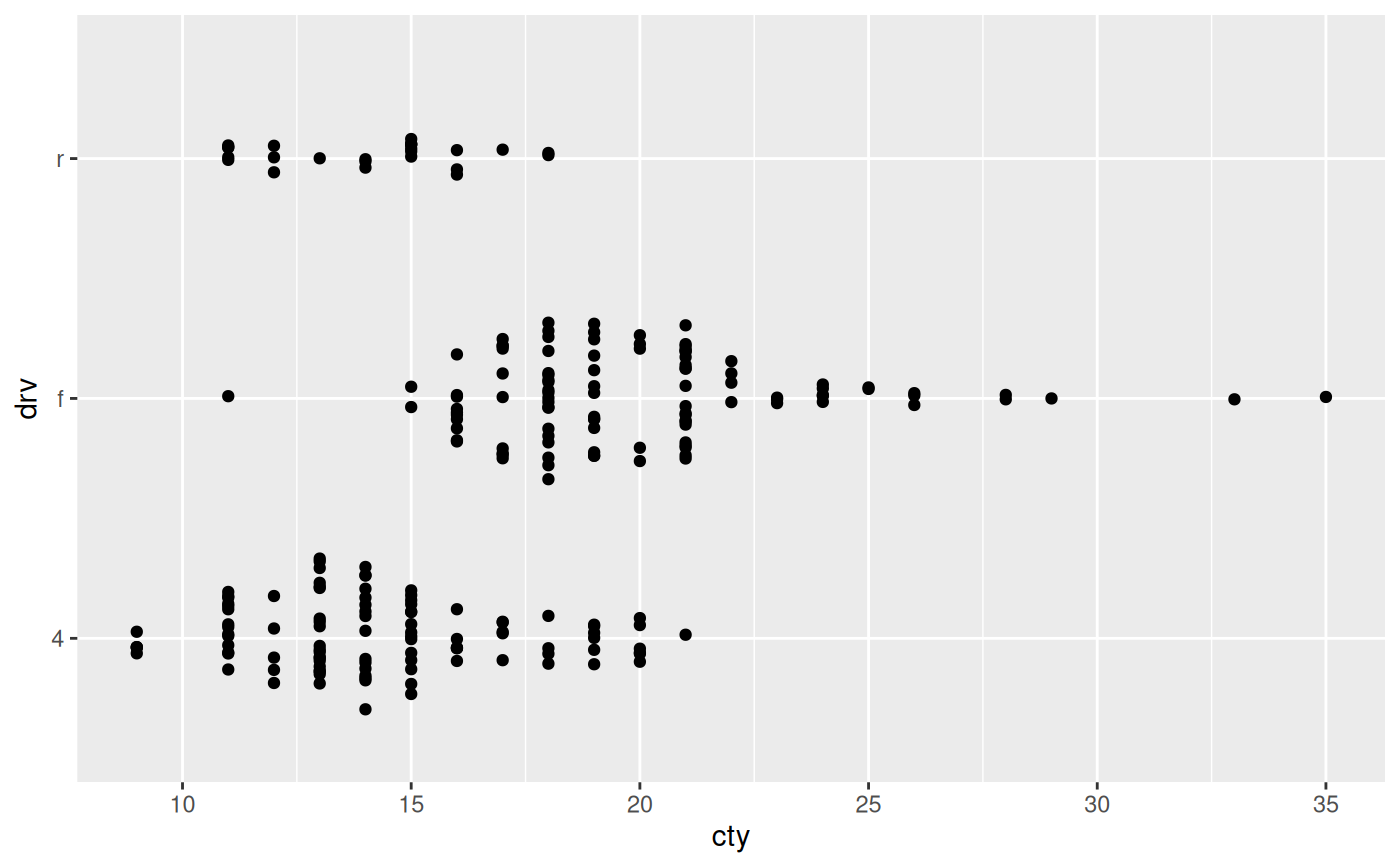# Discrete vs discrete: disc-jitter
ggplot(mpg) + geom_point(aes(fl, drv), position = 'auto')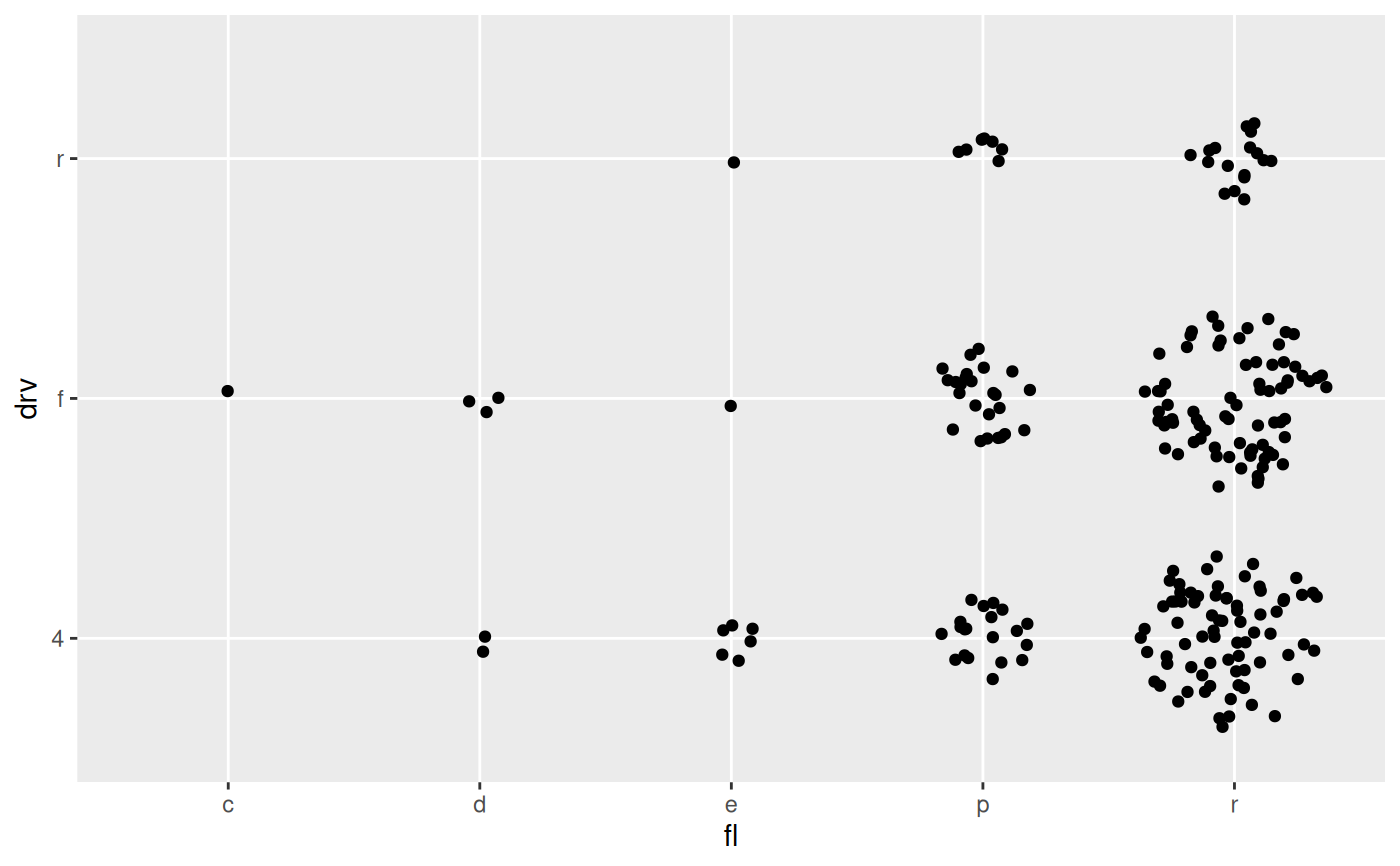# Don't scale the jitter based on group size
ggplot(mpg) + geom_point(aes(cty, drv), position = position_auto(scale = FALSE))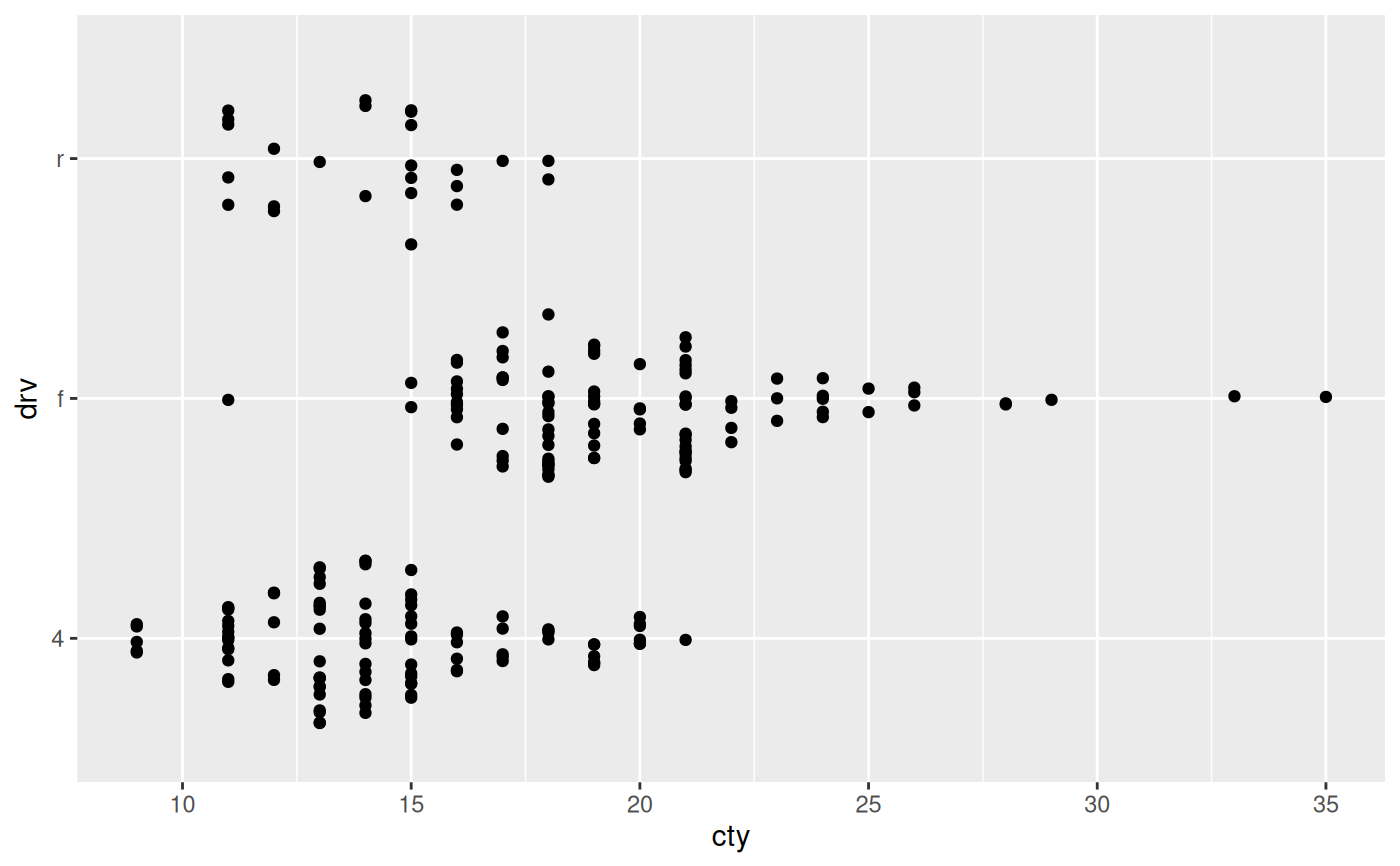ggplot(mpg) + geom_point(aes(fl, drv), position = position_auto(scale = FALSE))